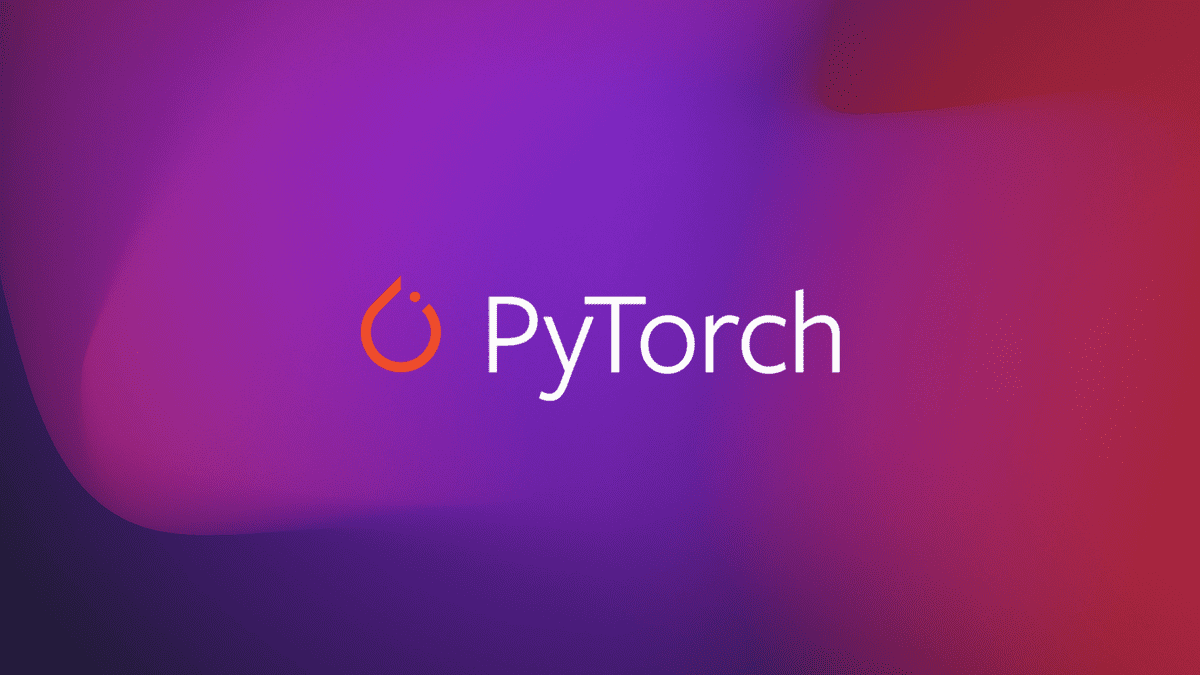# Insurance cost prediction using linear regression. 💡

Insurance cost prediction using linear regression.In this assignment we’re going to use information like a person’s age, sex, BMI, no. of children and smoking habit to predict the price of yearly medical bills. This kind of model is useful for insurance companies to determine the yearly insurance premium for a person. The dataset for this problem is taken from: https://www.kaggle.com/mirichoi0218/insurance We will create a model with the following steps: 1. Download and explore the dataset 2. Prepare the dataset for training 3. Create a linear regression model 4. Train the model to fit the data 5. Make predictions using the trained model This assignment builds upon the concepts from the first 2 lectures. It will help to review these Jupyter notebooks: PyTorch basics: https://jovian.ml/aakashns/01-pytorch-basics Linear Regression: https://jovian.ml/aakashns/02-linear-regression Logistic Regression: https://jovian.ml/aakashns/03-logistic-regression Linear regression (minimal): https://jovian.ml/aakashns/housing-linear-minimal Logistic regression (minimal): https://jovian.ml/aakashns/mnist-logistic-minimal

Let’s Start! Check out the original post on Medium.